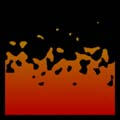### RSLFlames and Opacity

#### Introduction

This tutorial develops a self-luminescent surface shader that looks somewhat like a flame. The shader begins as a simple color ramp shader - listing 1.

Listing 1

 ```surface ramp(float Kfb = 1; color top = color(1,0,0), lower = color(1,1,0)) { color surfcolor = mix(top, lower, t); Oi = Os; Ci = Oi * Cs * surfcolor * Kfb; } ```#### Hard Edged Flames

Using `noise(p)`, where p is a transformed copy of the surface point `P`, a simple test can be applied to determine whether the 't' location of a micro-polygon is above or below a pre-defined noise value.Listing 2

 ```surface flame(float Kfb = 1, freq = 6; color top = color(1,0,0), lower = color(1,1,0); string spacename = "shader") { point p = transform(spacename, P); color surfcolor = mix(top, lower, t); if(t > noise(p * freq)) Oi = Os; else Oi = 0; Ci = Oi * Cs * surfcolor * Kfb; }```

#### Soft Edged Flames

Although the `if-else` statement is simple it causes aliasing. The problem stems from the way that opacity is assigned a value - its on the basis of a binary decision - on/off, true/false.

To soften the edges of the flames their opacity must be reduced gradually from the true `Os` surface opacity to zero. The `if-else` statements must be removed and replaced by the `smoothstep()` function. When converting an if-else "into" a smoothstep it is generally best to take the first part of the if statement and divide it into two values ie.

```       if(t > noise(p * freq))
/\
/  \
/    \
t-0.1,   t+0.1```

The two new values become the first parameters to the smoothstep function. The second part of the if-else statement becomes the final parameter to smoothstep ie.

```    if(t > noise(p * freq))
|
|________________________
|
Oi = 1 - smoothstep(t-0.1,t+0.1,noise(p * freq));```

Subtracting the value of smoothstep from 1.0 reverses the "dirction" of the flames. The animation shown below demonstrates the effects of softening the edges of the flame.

The final version of this shader uses a parameter called "soft" instead of an explicit value of 0.1.

#### Extensions

The techniques shown in this tutorial have only been applied to opacity. The color of the flame was created using the `mix()` function - effectively a simple color ramp. However, the color of the flames could either be created using noise or they could be based on the color ramp modified by a small "noisey" value.

Flames often show sparkle effects as a result of dust and other small particles passing through the flame. Bright white sparkles could be added to the flame by generating a high frequency noise() value. For example, the last line of shader code ie.

```    Ci = Oi * Cs * surfcolor * Kfb;
```

might be modified as,

```    if(high_frequency_noise > 0.7)
surfcolor = color(1,1,1);
Ci = Oi * Cs * surfcolor * Kfb;
```

How high_frequency_noise is calculated is left as a problem for the reader - refer to the tutorial " RSL: Writing Displacement Shaders".